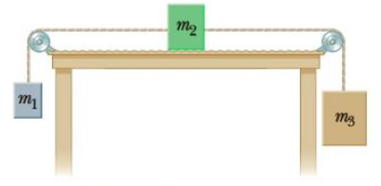Chapter 5, Problem 85AP

Chapter
Section
Textbook Problem

Three objects with masses m1 = 5.00 kg, m2 = 10.0 kg, and m3 = 15.0 kg, respectively, are attached by strings over frictionless pulleys as indicated in Figure P5.85. The horizontal surface exerts a force of friction of 30.0 N on m2. If the system is released from rest, use energy concepts to find the speed of m3 after it moves down 4.00 m.Figure P5.85

To determine
The speed of the mass m3 after it moves down 4.0m .

Explanation

Given Info:

The mass of m1 is 5.0kg .

The mass of m2 is 10.0kg .

The mass of m3 is 15.0kg .

The force of friction on m2 is 30.0N .

Since, the three masses are connected with string, when the mass m3 moves a distance d downward, the mass m2 will slide the same distance d to the right side on the horizontal top and the mass m1 will move a distance of d upward. Because all masses are connected with string the speed of all the masses will be the same.

According to Work-Energy theorem,

Wnc=ΔKE+ΔPEg

Thus,

fd=12(m1+m2+m3)(vf2vi2)+(m1g(d)+m2g(0)+m3g(d))

• f is the force of friction the table exerts on the mass m2
• vf is the final velocity of the masses
• vi is the initial velocity of the masses
• g is acceleration due to gravity

Formula to calculate the final velocity is,

Still sussing out bartleby?

Check out a sample textbook solution.

See a sample solution

The Solution to Your Study Problems

Bartleby provides explanations to thousands of textbook problems written by our experts, many with advanced degrees!

Get Started